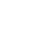Live chatAccording to the scatter plot diagram with the trend line, the trend line has a negative gradient. The negative gradient implies that if the number of earned run average (ERA) is more, then the team is not likely to win. For a team to win it has to try as much as possible to reduce the number of earned run average. A line of best fit is drawn to represent the data and it is clear that most teams tend to cluster below the line of best fit which is the trend line in this case. When the number of ERA is still small, the teams are scattered far apart but as the number of ERA increases they tend to cluster close to each other. It is evident that winning with less ERA is more difficult as compared to winning with more ERA thus the trend in the scatter diagram.

Considering the two hypotheses that were formulated, we have to reject the null hypothesis and adopt the alternative or the research hypothesis because there is a correlation however little it could be. When formulating a decision rule, we can reject the null hypothesis (H0) when the value of t is either greater than 2.467 or less than -2.467; and in our calculation the value of it is -5.45062. This means that we have to reject the null hypothesis and accept the alternate hypothesis because the value of t does not fall within the given range. The correlation that exists between the ERA and the number of wins is evident in the scatter plot diagram whereby as the ERA increases, the number of wins reduces and vise versa. In a summary, according to the information analyzed between a correlation exists between the number of wins and the ERA as mentioned above.

0

Preparing Orders

0

Active Writers

0%

Positive Feedback

0

Support Agents

Limited offerget 15% off your 1st order with code first15